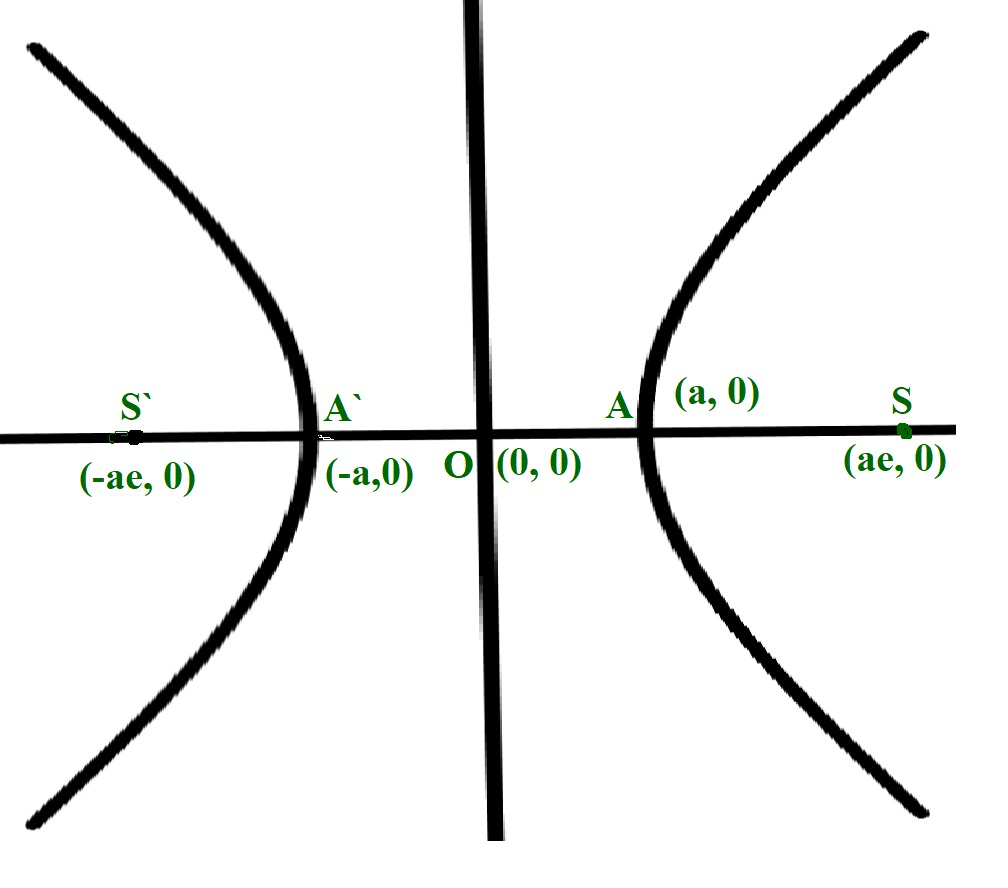Skip to content
Related Articles
Program to find the Eccentricity of a Hyperbola
• Last Updated : 13 May, 2021

Given two integers A and B, representing the length of the semi-major and semi-minor axis of a Hyperbola of the equation (X2 / A2) – (Y2 / B2) = 1, the task is to calculate the eccentricity of the given hyperbola.

Examples:

Input: A = 3, B = 2
Output: 1.20185
Explanation:
The eccentricity of the given hyperbola is 1.20185.

Input: A = 6, B = 3
Output: 1.11803

Approach: The given problem can be solved by using the formula to find the eccentricity of an ellipse.• The length of the semi-major axis is A.
• The length of the semi-minor axis is B.
• Therefore, the eccentricity of the ellipse is given bywhere A > B

Therefore, the idea is to print the value ofas the eccentricity of the ellipse.

Below is the implementation of the above approach:

## C++

 // C++ program for the above approach #include using namespace std; // Function to find the eccentricity// of a hyperboladouble eccHyperbola(double A, double B){    // Stores the squared ratio    // of major axis to minor axis    double r = (double)B * B / A * A;     // Increment r by 1    r += 1;     // Return the square root of r    return sqrt(r);} // Driver Codeint main(){    double A = 3.0, B = 2.0;    cout << eccHyperbola(A, B);     return 0;}

## Java

 // Java program for the above approachimport java.util.*; class GFG{ // Function to find the eccentricity// of a hyperbolastatic double eccHyperbola(double A, double B){         // Stores the squared ratio    // of major axis to minor axis    double r = (double)B * B / A * A;     // Increment r by 1    r += 1;     // Return the square root of r    return Math.sqrt(r);} // Driver Codepublic static void main(String[] args){    double A = 3.0, B = 2.0;         System.out.print(eccHyperbola(A, B));}} // This code is contributed by Amit Katiyar

## Python3

 # Python3 program for the above approachimport math # Function to find the eccentricity# of a hyperbola  def eccHyperbola(A, B):     # Stores the squared ratio    # of major axis to minor axis    r = B * B / A * A     # Increment r by 1    r += 1     # Return the square root of r    return math.sqrt(r)  # Driver Codeif __name__ == "__main__":     A = 3.0    B = 2.0    print(eccHyperbola(A, B))     # This code is contributed by ukasp

## C#

 // C# program for the above approachusing System; class GFG{ // Function to find the eccentricity// of a hyperbolastatic double eccHyperbola(double A, double B){         // Stores the squared ratio    // of major axis to minor axis    double r = (double)B * B / A * A;     // Increment r by 1    r += 1;     // Return the square root of r    return Math.Sqrt(r);} // Driver Codepublic static void Main(String[] args){    double A = 3.0, B = 2.0;         Console.Write(eccHyperbola(A, B));}} // This code is contributed by Princi Singh

## Javascript

 
Output:
2.23607

Time Complexity: O(1)
Auxiliary Space: O(1)

Attention reader! Don’t stop learning now. Get hold of all the important DSA concepts with the DSA Self Paced Course at a student-friendly price and become industry ready.  To complete your preparation from learning a language to DS Algo and many more,  please refer Complete Interview Preparation Course.

In case you wish to attend live classes with industry experts, please refer DSA Live Classes

My Personal Notes arrow_drop_up